Next: Vertex Fitting with Error Up: Vertex Fitting Previous: Vertex Fitting

## Vertex Fitting without Error Matrix

In order to supply an initial vertex position, we first calculate an approximate vertex position by calculating the intersection in the xy plane of two helices arbitrarily chosen from the given set of tracks. There are two such intersections in general. We then compares the differences of z coordinates and take the intersection corresponding to the smaller distance. The mid-point in the z direction is our first guess of the common vertex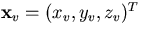.We approximate the helices to tangential lines at the points on the helices that are the closest in the xy plane to the trial vertex position: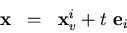(26)
where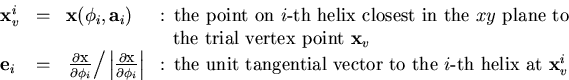(27)
and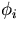is given by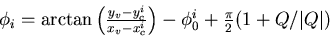(28)
with (xci,yci) being the center of the i-th helix: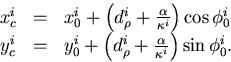(29)
Then, what to minimize is the sum of the distance squared of each tangential line from a new trial vertex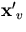: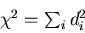(30)
with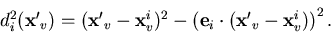(31)
Notice that the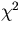is a quadratic funciton ofso that the minimization condition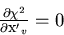(32)
is a linear equation which can be solved by a single matrix inversion. The solutionis our improved guess of the common vertex. We repeat this process until the vertex position converges.Next: Vertex Fitting with Error Up: Vertex Fitting Previous: Vertex Fitting
Keisuke Fujii
12/4/1998